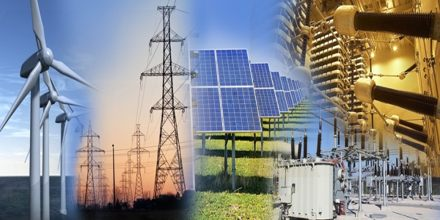Physics

# Electrical Powermajor purpose of this lecture is to present on Electrical Power. The instantaneous electrical power P delivered to a component is given by P(t) = V(t).I(t); where P(t) is the instantaneous power, measured in watts (joules per second). The electric power in watts associated with a complete electric circuit or a circuit component represents the rate at which energy is converted from the electrical energy of the moving charges to some other form, e.g., heat, mechanical energy, or energy stored in electric fields or magnetic fields.